We decided to build this topic to show you how mathematics is related to music. Maybe you don’t like math, but don’t worry, we will try to explain each concept in a simple way, so that you realize that our sensitivity to sound is linked to the logic of our brains. This is very interesting, so let go of your prejudices. All knowledge is nice when well taught.

Very well, in the first topics here in the website, we mentioned that sound is a wave and that the frequency of the sound is what defines the musical note.

But what is frequency? It is a repetition with time reference. Imagine a bicycle wheel spinning. If this wheel completes one revolution in 1 second, we say that the frequency of that wheel is “one revolution per second”, or “one Hertz”.

Hertz is just a name given to represent the unit of frequency, and is often abbreviated to “Hz”. If this wheel in our example completed 10 revolutions in 1 second, its frequency would be 10 Hertz (10 Hz).

Great, but what does that have to do with sound? Well, sound is a wave, and that wave oscillates with a certain frequency. If a sound wave completes one oscillation in 1 second, its frequency will be 1 Hz. If a sound wave completes 10 oscillations in 1 second, its frequency will be 10 Hz. For each frequency, we have a different sound (a different note). The A note, for example, corresponds to a frequency of 440 Hz.

And where does mathematics come into play here? It has been observed that when a frequency is multiplied by 2, the note remains the same. For example, the A note (440 Hz) multiplied by 2 = 880 Hz is also an A note, just one octave above.

If the goal was to lower an octave, it would be enough to divide it by 2. We can then conclude that a note and its respective octave maintain a ratio of ½.

## Pythagoras’ experiments

Okay, so before we continue, let’s go back to the past, to Ancient Greece. At that time, there was a man named Pythagoras who made very important discoveries for mathematics (and for music). This we have just shown about octaves, he discovered while “playing” with a taut string.

Imagine a stretched rope, attached to its ends. When we play this string, it vibrates (see the drawing below):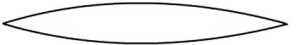Pythagoras decided to divide this string into two parts and touched each end again. The sound produced was exactly the same, only higher (since it was the same note an octave higher):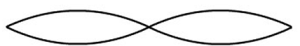Pythagoras did not stop there. He decided to try what the sound would look like if the string was divided into 3 parts: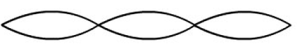He noticed that a new sound appeared, different from the previous one. This time, it was not the same note an octave higher, but a different note, which needed to be renamed. This sound, despite being different, combined well with the previous sound, creating a pleasant harmony to the ear, because these divisions shown until now have 1/2 and 2/3 mathematical relationships (our brain likes well-defined logical relationships).

So, he continued making subdivisions and mathematically combined the sounds creating scales that later stimulated the creation of musical instruments that could reproduce these scales.

The tritone interval, for example, was obtained from the 32/45 ratio, a complex relationship, a factor that leads our brain to consider this sound unstable and tense.

Over time, the notes were given the names we know today.

Interestingly enough, in general, the human brain interprets sounds as “pleasant” from small values in the numerator and denominator of a fraction, such as the 2/3, 4/5, 8/5, etc. The fraction 32/45 sounds “unpleasant”.

Although there is no scientific evidence to justify this, the reason may be the combination of periods, where very misaligned periods are more difficult to interpret. For example, imagine a sound that plays every two seconds, along with another sound that plays every 3 seconds (resulting in a 2/3 fraction). The pattern resulting from this combination of two rhythms could be quickly identified. However, two sounds that played in the proportions of 32 by 45 would form a rhythmic pattern more difficult to decipher. If this idea is not very clear, consider the following:

In practice, as we have already seen, a musical note is formed by beats played quickly in succession (for example: 220 beats per second = 220 Hz).

When we play two notes at the same time, we are comparing a sound that hits X times per second with another that hits Y times per second, resulting in a X/Y fraction.

If the smallest form of this fraction results in small numbers, it means that the rhythmic pattern can be more easily interpreted.

In other words, each note has an associated rhythm, and the human brain interprets these rhythms in a range of pitch (high or low). The overlap of two notes, in their fundamental physical essence, is a rhythmic overlap. If the resulting rhythm consists of a simple and identifiable pattern, the sound interpretation is more pleasant.

## The mathematics of musical scalesMany peoples and cultures have created their own musical scales. An example was the Chinese people, who started from the experience of Pythagoras (using ropes). They played the C note on a stretched string and then divided that string into 3 parts, as we just showed.

The result of this division was the G note. When observing that these notes had a harmony with each other, they repeated the procedure from that G note, again dividing this piece of string into 3 parts, resulting in the D note. This note had a pleasant harmony with the G note and also with the C note.

This procedure was then repeated from the D note, giving rise to the A note. Then, starting from A, we reached the E note.

When they repeated this procedure of dividing the string into 3 parts once again, giving rise to the B note, there was a problem, as the B note did not sound very well when played together with the C note (the first note of the experiment). In fact, these notes were very close to each other, which caused some discomfort in the sound.

For this reason, the Chinese ended their divisions by obtaining the C, G, D, A and E notes, leaving the B note aside. These notes served as the basis for Chinese music, forming a 5-note scale (Pentatonic). That pentatonic scale, due to being pleasant and consonant, represented the oriental culture very well, which has always been guided by harmony and stability.

Since its creation until today, the pentatonic scale represents a great option for melodies, as we have already mentioned in the pentatonic scale article. But let’s go back to the subject of notes and frequencies, after all we have only shown 5 notes on the scale so far.

Western music, which works with 12 notes, did not discard the B note as oriental culture had done. Westerners noted that the C and B notes were close to each other and decided to create a more comprehensive scale. On this scale, all notes should be the same distance from each other. And that distance should be the interval between C and B (one semitone). That is, between C and D, for example, there would need to be an intermediate note, because the distance between C and D (two semitones) was greater than the distance between C and B (one semitone). Through the analysis of frequencies, it was discovered that by multiplying the frequency of the B note by the number 1.0595 we would get the frequency of the C note:

• B note frequency: 246,9 Hz
• C note frequency: 261,6 Hz

By multiplying the frequency of the B note by 1.0595, we have:

246.9 x 1.0595 = 261.6 Hz (C note)

Since our goal is to maintain this same relationship (distance) to the other notes, we will use this procedure to find out which note will come after C. By multiplying the frequency of the C note by 1.0595, we have:

261.6 x 1.0595 = 277.2 Hz (C sharp note)

Repeating this procedure to see what comes after C sharp:

277.2 x 1.0595 = 293.6 Hz (D note)

Notice that following this logic, we can form the entire chromatic scale! That is, after multiplying the frequency of the C note by the number “1.0595” twelve times, we will return to the C note. This is only possible because “1.0595” corresponds to the result of the root.

Note that the root multiplied by itself 12 times is ()12 = 2.

And we have already seen that a note multiplied by 2 is itself an octave higher.

Now we can clearly see that these numbers are not by chance. The objective from the beginning was to divide a scale into 12 equal parts, so that the last note was once again the first. That’s how the tempered scale, also called the chromatic scale, came about.

## By finding notes from frequencies

Everything we discussed will become clearer by looking at the notes on a piano:If we consider that the first C (leftmost) has an f frequency, the second C (one octave higher will have a 2f frequency). To get to the next C, we will have to take C 2f and multiply by 2 once again, reaching C 4f. By repeating the process, the last C of that piano will be C 8f. Follow the logic: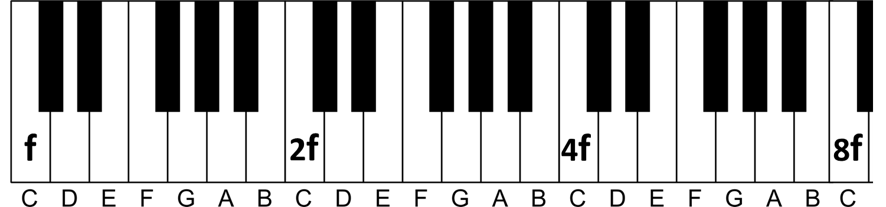But what about frequencies 3f, 5f, 6f and 7f, where they are? Let’s find out. First let’s think about frequency 3f. It is somewhere between frequencies 2f and 4f. We know that 1.5*2 = 3, so we need to advance a k amount of semitones (where each semitone equals approximately 1.0595, as we have seen) until it results in 3f. That is, (1.0595^k)*2f = 3f. Solving for k, we find that this value is 7, since 1.0595^7 = 1.5 (approximately).

In other words, we need to advance 7 semitones from 2f until you reach note 3f. The conclusion is that note 3f is the G note: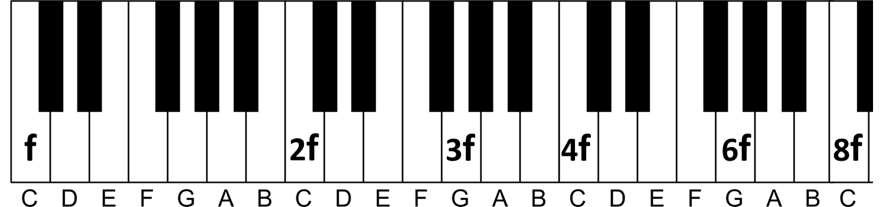Note that we can now automatically conclude where note 6f is because it will be one octave above 3f: 3f*2 = 6f. That is why it has also been identified above.

By performing the same procedure, we can find note 5f, which is halfway between frequencies 4f and 6f: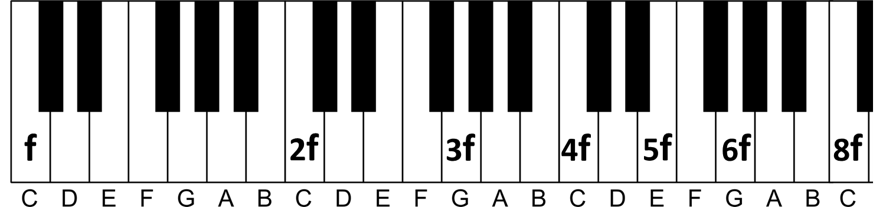Now we can also think backwards to find the frequencies of the first octave. So far we have always advanced by multiplying by 2 to reach the highest notes. If we want to find the lowest notes, just do the reverse process: divide by 2.

That is, the “E” note (mi) denoted as 5f located one octave below will be (5/2)*f. And by reducing one more octave, it will be (5/4)*f. The same goes for the 3f note: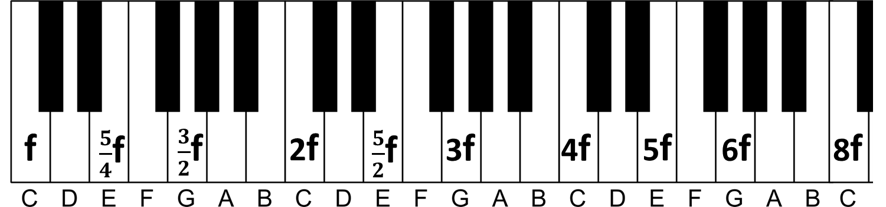Now everything starts to become clearer. The 5/4 and 3/2 relationships that we started talking about in this article when we mentioned the Pythagoras experiment are materializing on our piano.

Using the same techniques discussed so far, we can accurately establish all fractions of the first octave: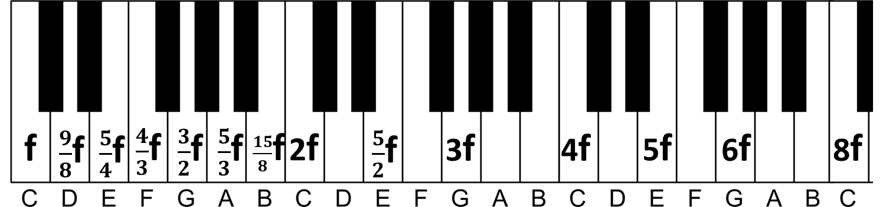To find out if two notes played at the same time will sound pleasant, just divide your fractions and reduce the expression to its most basic form. If the result is a large value in the denominator, the feeling will be unpleasant.

### Examples:

The interval between E and B corresponds to a perfect fifth. Perfect fifth intervals are very pleasant musically speaking. Can we verify this mathematically? Just do (5/4)/(15/8) = 40/60 = 2/3. Simple fraction (small denominator), as expected.

And the interval between B and C? There is a sound clash between B and C (notes separated by a semitone apart do not sound well together). Let’s see what mathematics tells us about this interval: (15/8) / (2/1) = 15/16. Complex fraction, as expected.

## Logarithm in Music

If you understand a bit of mathematics you noticed that, when doing the calculations of frequencies and roots, we work intrinsically with the logarithm to the base 2. For this reason, piano builders placed the shape of a logarithm chart on the body of the piano, to make a reference to this mathematical-musical discovery. Observe:

Example logarithmic graph: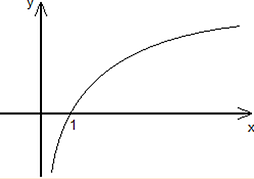Piano body: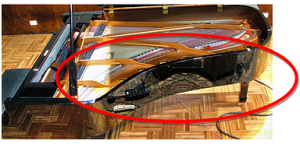## Going Further

There are many other mathematical explanations for various questions in music, and to show them here it will be necessary to address more advanced subjects, such as the Fourier series. A Fourier series can be used to describe the behavior of a wave in physics. Basically, this series is formed by a main harmonic added to other secondary harmonics. The equation can be described as follows: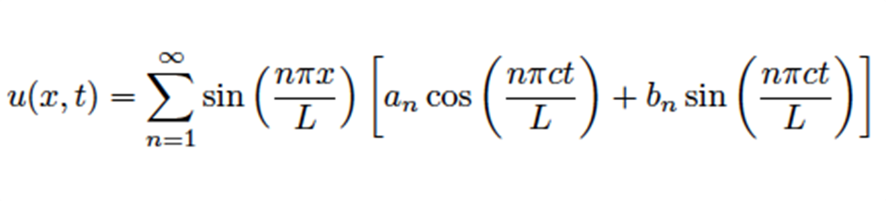When a string vibrates, what we hear is not a single pure sound, but a superposition of several sounds, whose frequencies are all multiples of the fundamental frequency. The fundamental frequency is the main one, which contributes the most, and the other multiple frequencies are called harmonics.

The amount of harmonics adds “richness” to the sound, giving it body. The sound of a tuning fork, for example, does not have the highest harmonics, basically containing only the fundamental frequency. This is great as a reference (for this reason the tuning fork is usually used to tune instruments), but in contrast, the tuning fork has a poor sound, without much beauty or richness of sound.

The proportion with which harmonics are added to a fundamental frequency contributes to the production of timbre characteristic of each instrument. This is why the harmonica has a different sound than the flute, for example.

And obviously, different materials produce different harmonics. This means that the production of a quality instrument takes into account each characteristic of each material, such as the type of wood used in the body of a guitar.

Going a step further, when a musician plugs an instrument through a cable to the amplifier, each element in this circuit may end up filtering some harmonics, which reduces the sound quality. This is why it is very important to invest not only in an instrument, but in each particular equipment.

The sound engineering process, which develops analog-digital devices to capture sound waves and store them digitally, always seeks to preserve the original wave shape as much as possible. Studio editing such as noise removal also use these concepts, trying to identify which harmonics are polluting the original wave.

## Final Thoughts

Our goal was to show you how music works mathematically and how logical relationships are understood by our brain.

Obviously, we did everything here using approximations (rounded numbers), as a more accurate analysis would be tedious for most readers and would also require more rigorous mathematical and physical testing.

It is not necessary to memorize everything we teach in this topic, just keep in mind that music did not come out of nowhere, it is the result of a numerical organization. All of this interpretation is done by our brains.

The bottom line is that if you are a musician, then you are (in one way or another) a mathematician, as the feelings of pleasure you feel when listening to music hide subliminal calculations.

Your brain likes calculations, it’s a calculating machine! The more you practice, study and know music, the more this skill will develop. You will probably start to enjoy listening to music that did not bring you great feelings before.

We can compare this with a physics student from the 1st semester. If he reads a modern physics book, it will look like Greek to him; it will not bring him any pleasure. But a few years later, when he has reached a solid mathematical foundation and comes across this same book, perhaps he will come to love this subject and want to dedicate his life to it.

Go to: Module 12

Back to: Simplifying Theory